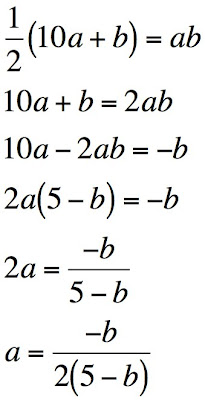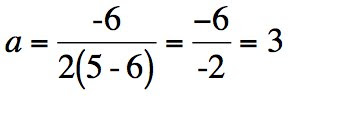## Tuesday, October 02, 2007

### MathNotations: Products of Digits: Challenges for Everyone...: Part 2

Remember Dave Marain's Challenge? I posted this challenge a couple of days ago here. He quoted a riddle from a book, The Righteous Men by Sam Bourne:

Just men we are, our number few
Describable in digits two
We're halved if these do multiply
If we few perish then all must die.

Dave then posed a challenge:
(1) Determine a 2-digit positive integer the product of whose digits is one-half the integer. Now, it won't take you long to find such a number (once you get past the elliptical phrasing), but that's just to whet your appetite. The real challenge begins:

(2) Prove that your answer to (1) is unique, i.e., there is only one solution to the problem.
Here is my solution:

Since the solution is a two digit number, we have two "slots" to fill, one for the tens digit and one for the ones digit. The digit in the tens place will be multiplied by 10 and the digit in the ones place will be multiplied by 1.

I will call the first digit, in the tens place, a; and I call the 2nd digit, in the ones place, b.Because both a and b must be positive integers (we cannot have parts of people or negative amounts of people), we can only consider positive values of b. We are also restricted to the numbers 1 through 9 because we can only use one digit in each place value location.

In order for the expression for the value of a to be positive, 2b must be greater than 10 (or, b must be greater than 5). This leaves us with only the numbers 6, 7, 8 and 9 to consider.

We can now eliminate the odd numbers (7 and 9) because the numerator of the expression for the value of a must be an even number because the denominator will always be even (because the difference of 5 and b is always doubled). If the denominator will always be even, then the numerator must be even in order to get an integer.

We are now left with only 6 and 8 as possible solutions for b:

If b = 6:If b = 8:Therefore, 6 is the only possible solution for b. If b = 6, a = 3. Therefore, the integer we are looking for is 36. Half of thirty-six is 18. The product of 3 and 6 is 18. There were 36 people.

My middle school students would undoubtedly solve this problem using a table in Excel. They have had it drilled into them to show three ways to solve a problem: table, graph and equation. In this case, the graph would not be a proof for the solution but it would be confirmation that their solution is correct (assuming that their calculations are correct). I am curious about what equation they would be able to come up with, if any.In the chart above, I failed to skitch in the point where both graphs intersect. But our students know how to explore the Excel chart to find where they intersect. As expected, the graph reflects the table from which it was made.

I am considering giving them this problem as one of their portfolio problems. I hope I have proof-read the first solution so that it is clear and error-free.

Technorati tags:

_/\_/\_

#### 1 comment:

1.Hi Meeyauw!
I really appreciate your sharing this problem with your middle school students. Your proof was very nice and similar to the way I did it. It's interesting how many variations there are, each one depending on the FORM of the equation used for the analysis.

I am particularly impressed by the multiple representation approach your students have become accustomed to using. This is phenomenal! Using the power of Excel (particularly its graph capability) in a math class is well-known to some educators and fairly unknown to others.

My thoughts about proof at this level are that just as adolescents show extreme variations in physical characteristics in that age group, there is also a wide variation in their mathematical maturity. Some middle schoolers I've worked with (7th graders) are ready for proof and can even do them on their own after seeing a few models. For awhile I listened to 'experts' explain that a formal geometry course is inappropriate for a middle schooler because of immature brain development, meaning they are just not ready to handle the conceptualizations. Perhaps not many, but some can, and I'm not just referring to a few mathematical geniuses out there. I'll bet if you showed your students your proof and explained the main ideas, some would be able to follow and a few, if they could handle the algebra skill part, could do a similar one on their own. Hey, you never know until you try and I know that you are not afraid to take risks.

Using my problems/investigations with your students make writing these worthwhile. Thank you and let me know how it goes with that portfolio assignment.

Dave Marain
MathNotations